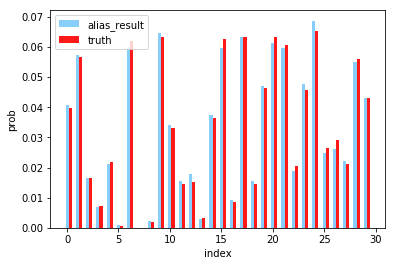## O(N)的方法

1. 生成01均匀分布2. 查找下标k使得3. 返回k

## O(1)的方法

Alias方法将整个概率分布压成一个的矩形，对于每个事件，转换为对应矩形中的面积为## Python代码

import numpy as np
def gen_prob_dist(N):
p = np.random.randint(0,100,N)
return p/np.sum(p)

def create_alias_table(area_ratio):

l = len(area_ratio)
accept, alias =  * l,  * l
small, large = [], []

for i, prob in enumerate(area_ratio):
if prob < 1.0:
small.append(i)
else:
large.append(i)

while small and large:
small_idx, large_idx = small.pop(), large.pop()
accept[small_idx] = area_ratio[small_idx]
alias[small_idx] = large_idx
area_ratio[large_idx] = area_ratio[large_idx] - (1 - area_ratio[small_idx])
if area_ratio[large_idx] < 1.0:
small.append(large_idx)
else:
large.append(large_idx)

while large:
large_idx = large.pop()
accept[large_idx] = 1
while small:
small_idx = small.pop()
accept[small_idx] = 1

return accept,alias

def alias_sample(accept, alias):
N = len(accept)
i = int(np.random.random()*N)
r = np.random.random()
if r < accept[i]:
return i
else:
return alias[i]

def simulate(N=100,k=10000,):

truth = gen_prob_dist(N)

area_ratio = truth*N
accept,alias = create_alias_table(area_ratio)

ans = np.zeros(N)
for _ in range(k):
i = alias_sample(accept,alias)
ans[i] += 1
return ans/np.sum(ans),truth

if __name__ == "__main__":
alias_result,truth = simulate()